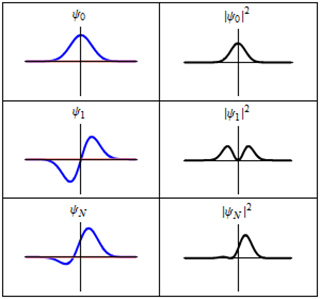Course Description
In this course, we study elliptic Partial Differential Equations (PDEs) with variable coefficients building up to the minimal surface equation. Then we study Fourier and harmonic analysis, emphasizing applications of Fourier analysis. We will see some applications in combinatorics / number theory, like the Gauss circle …
Course Info
Instructor
Departments
Topics
Learning Resource Types
assignment Problem Sets
notes Lecture NotesEach of these three rows is a wave function which satisfies the time-dependent Schrödinger equation for a harmonic oscillator. (This image is in the public domain. Original animated image by Sbyrnes321 on Wikipedia.)